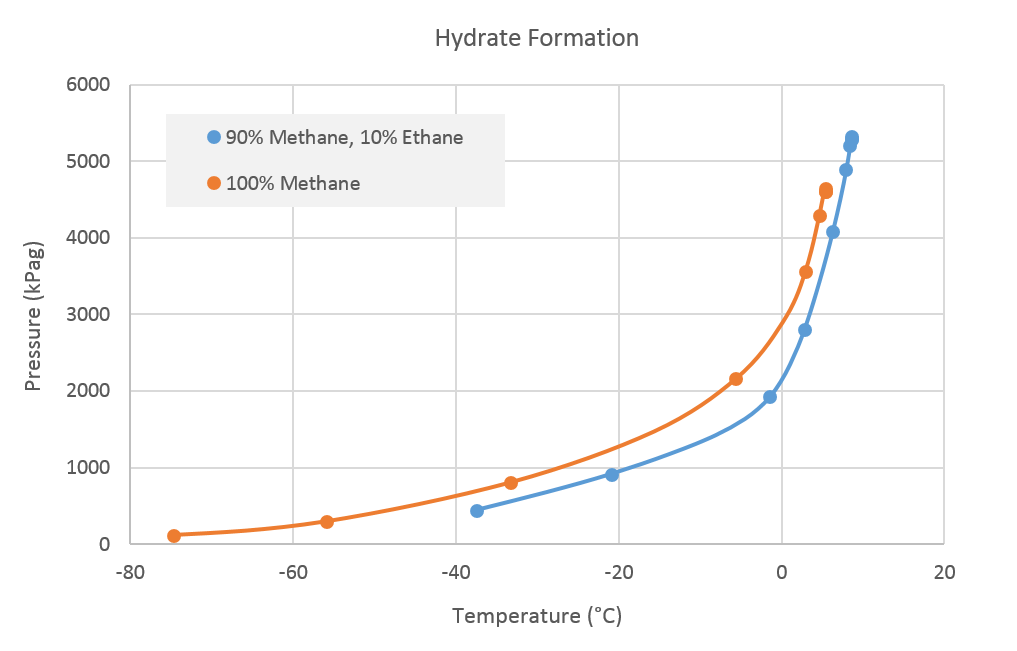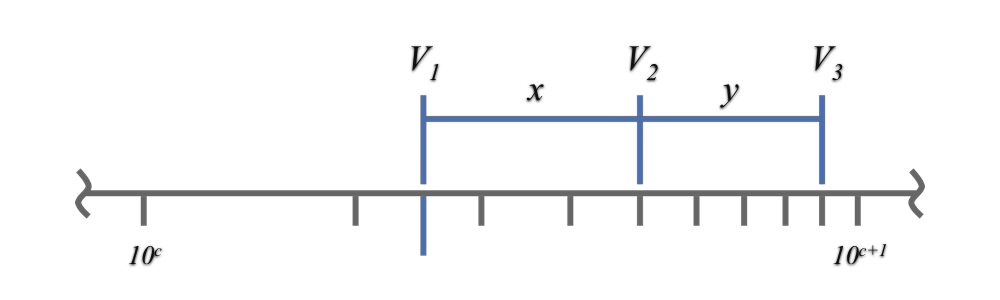f
Neutrium

# General Engineering

Index of Dimensionless Numbers

Dimensionless numbers play an important role in analysing fluid dynamics and heat and mass transfer problems. They provide a method by which complex phenomena can be characterised, often by way of a simple, single number comparison. This article provides a summary of dimensionless numbers and the formulae used to calculate them.

Dimensionless Numbers and Dimensional Analysis

Dimensionless numbers play an important role in analysing fluid dynamics and heat and mass transfer problems. They provide a method by which complex phenomena can be characterised, often by way of a simple, single number comparison. This article provides a brief overview of the derivation and use of dimensionless numbers.

Episode 1 - Unit ConversionSince the dawn of thinking, mankind has been measuring things. If humans are going to tell each other about all the great counting and measuring they have been doing, they need to put it in terms that others can understand. In Neutrium’s first episode, Trevor and Matthew discuss unit conversions.

Hydrate Formation in Gas SystemsHydrate formation represents a significant risk to process safety as it can result in the plugging of both pipes and instruments. Hydrates typically form in process where light hydrocarbons, water vapor and low temperatures or high pressures are present. This article describes the conditions under which hydrates form, how formation may be prevented and what can be done once hydrates have formed.

Baumé Scale

The Baumé scale was first developed in 1768 as a method of measuring the density of liquids. Today it is largely superseded, however it is still used in some industries as a measure of density or concentration. This article presents formula for the calculation of degrees Baumé for a liquid.

API Gravity

API gravity is measure of the density of liquid hydrocarbons relative to water. This article provides a reference to the API gravity calculation formula and some typical values of API gravity for common hydrocarbons.Reading values from a graph or plot with a logarithmic axis visually is difficult and will often result in inaccurate readings. This article presents formulae which may be used with measurements from a graph axis to obtain more accurate values.

Nominal Pipe Characteristics in Metric Units

Nominal Pipe Size (NPS) is a defined set of standard pipe sizes commonly used for process piping. NPS piping is defined in terms of a nominal diameter and wall thickness (defined by the pipe schedule). This article provides characteristics for NPS piping in metric units.

Nominal Pipe Characteristics in Imperial Units

Nominal Pipe Size (NPS) is a defined set of standard pipe sizes commonly used for process piping. NPS piping is defined in terms of a nominal diameter and wall thickness (defined by the pipe schedule). This article provides characteristics for NPS piping in Imperial units.